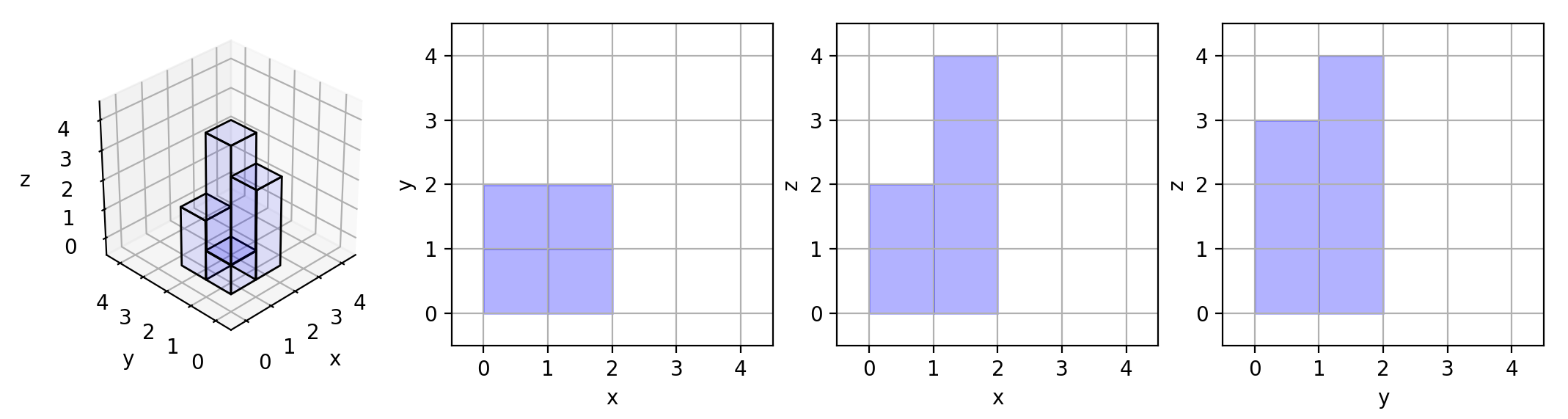# LeetCode: 887. 三维形体投影面积¶

## 1、题目描述¶

N * N 的网格中，我们放置了一些与 x，y，z 三轴对齐的 1 * 1 * 1 立方体。

输入：[]



输入：[[1,2],[3,4]]输入：[[1,0],[0,2]]



输入：[[1,1,1],[1,0,1],[1,1,1]]



输入：[[2,2,2],[2,1,2],[2,2,2]]



• 1 <= grid.length = grid.length <= 50
• 0 <= grid[i][j] <= 50

## 2、解题思路¶

​ 首先来分析一下，不同的平面的面积该如何计算

• xy底面投影

• 对于每一个有方块的坐标，只要不为0，面积加一

• 例如

• [[1,1,1],[1,0,1],[1,1,1]] 面积就是不为0的个数，也就是8

• xz对面投影

• 对于正前方的投影，对于每一个x坐标相同的，取最大值即可

• 例如

• [[1,0],[0,2]] 每一次取最大值，就是1+2=3

• yz左面投影

• 对于左面投影，这个是求解当y相同的时候，取出最大的那个

• 例如

• [[2,2,2],[2,1,2],[2,2,2]] 相当于每一次取出每一个元素的对应位置的元素，取出最大的，然后加起来 2+2+2=6

python class Solution: def projectionArea(self, grid): """ :type grid: List[List[int]] :rtype: int """

    area_zy = sum([1 if i else 0 for pos in grid for i in pos])
area_xz = sum([max(pos) for pos in grid])
area_yz = sum([max(p) for p in zip(*grid)])
return area_zy + area_xz + area_yz


​ 上面的情况都是横坐标中，对应的list长度都一致，如果不一致，在求解yz的时候，就需要使用zip_longest，而不是zip，如下所示：python import itertools class Solution: def projectionArea(self, grid): """ :type grid: List[List[int]] :rtype: int """ area_zy = sum([1 if i else 0 for pos in grid for i in pos]) area_xz = sum([max(pos) for pos in grid]) area_yz = sum([max(p) for p in itertools.zip_longest(*grid, fillvalue=0)]) return area_zy + area_xz + area_yz